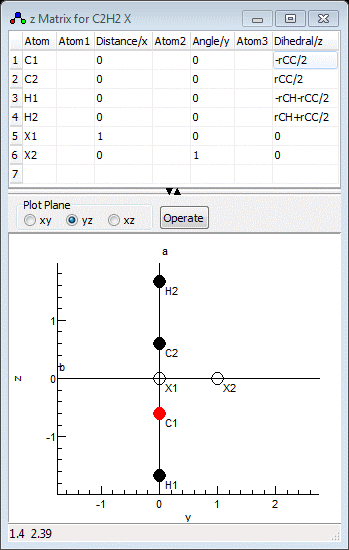Molecule Types Vibrational Structure Force Field Analysis Samples

# Force Field Analysis for Acetylene

The files listed below reproduce the fits given in Table 1a and Table 1b of L. Hedberg and I. M. Mills,  J. Molec. Spectrosc. 203, 82 (2000). The files are:

• C2H2ff.obs - the observed quantities for the fit for use with either of the files below. Points to note:
• Several different (equivalent) ways are used to specify the parameters.
• The 13C vibrational frequencies are fitted as isotope shifts, as these are typically more accurate than absolute values.
• Different weights (estimated errors ) are used for the observed values for C2D2, in line with the original paper.
• C2H2emp.pgo - a fit to purely empirical symmetry force constants.
• C2H2scaled.pgo - a fit scaling ab initio force constants to match experiment.

Notes on the data files:

• The force constants are expressed in symmetry co-ordinates, rather than internal valence coordinates.
• The two degenerate bending modes are repeated with the same force constant, so F4 and F6 appear twice in the force constant list.
• The z matrix, shown below, uses Cartesian coordinates to specify the atomic positions, and has two dummy atoms to allow the bending modes to be uniquely specified.